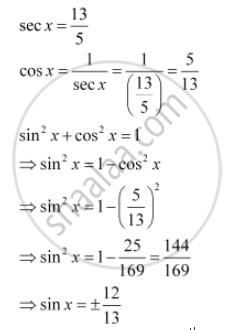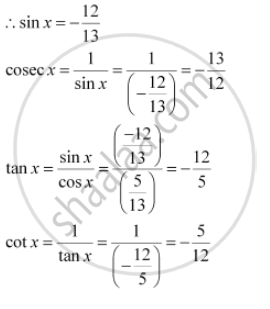CBSE (Arts) Class 11CBSE
Share

# Find the Values of Other Five Trigonometric Functions If Sec X = 13/5 , X Lies In Fourth Quadrant. - CBSE (Arts) Class 11 - Mathematics

ConceptSigns of Trigonometric Functions

#### Question

Find the values of other five trigonometric functions if  sec x = 13/5 , x  lies in fourth quadrant.

#### SolutionSince x lies in the 4th quadrant, the value of sin x will be negative.Is there an error in this question or solution?

#### APPEARS IN

NCERT Solution for Mathematics Textbook for Class 11 (2018 to Current)
Chapter 3: Trigonometric Functions
Q: 4 | Page no. 63

#### Video TutorialsVIEW ALL 

Solution Find the Values of Other Five Trigonometric Functions If Sec X = 13/5 , X Lies In Fourth Quadrant. Concept: Signs of Trigonometric Functions.
S